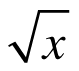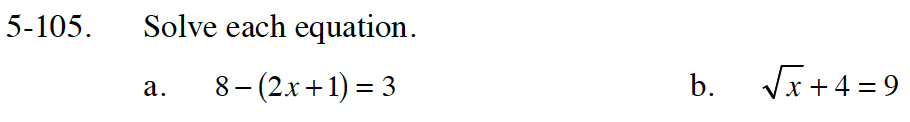Home > CC4 > Chapter 5 > Lesson 5.3.2 > Problem5-105

5-105.
1. Solve each equation. Homework Help ✎

1. 8 – (2x + 1) = 3

2.+ 4 = 98 − 2x − 1 = 3

7 − 2x = 3

− 2x = −4

Subtract four from both sides of the equation.

Square both sides of the equation.